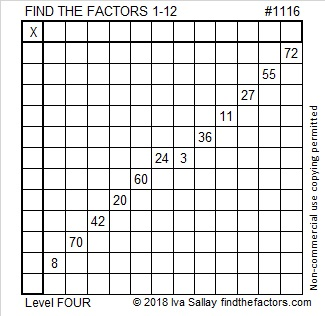# 1116 Can I Trick You With This Puzzle?

This is only a Level 4 puzzle, but can I trick you into making some assumptions about the puzzle’s factors? I bet I will trick a least a few people. Will you be one of them? If you don’t write a factor unless you are 100% sure it goes where you are putting it, I shouldn’t be able to trick you. Good luck with this one!Print the puzzles or type the solution in this excel file: 12 factors 1111-1119

Now I’ll share some facts about the number 1116:

• 1116 is a composite number.
• Prime factorization: 1116 = 2 × 2 × 3 × 3 × 31, which can be written 1116 = 2² × 3² × 31
• The exponents in the prime factorization are 2, 2 and 1. Adding one to each and multiplying we get (2 + 1)(2 + 1)(1 + 1) = 3 × 3 × 2 = 18. Therefore 1116 has exactly 18 factors.
• Factors of 1116: 1, 2, 3, 4, 6, 9, 12, 18, 31, 36, 62, 93, 124, 186, 279, 372, 558, 1116
• Factor pairs: 1116 = 1 × 1116, 2 × 558, 3 × 372, 4 × 279, 6 × 186, 9 × 124, 12 × 93, 18 × 62 or 31 × 36
• Taking the factor pair with the largest square number factor, we get √1116 = (√36)(√31) = 6√31 ≈ 33.406591116 looks interesting when it is represented in these other bases:
It’s 13431 in BASE 5 because 1(5⁴) + 3(5³) + 4(5²) + 3(5) + 1(1) = 1116,
VV in BASE 35 (V is 31 base 10) because 31(35) + 31(1) = 31(36) = 1116, and
V0 in BASE 36 because 31(36) = 1116

This site uses Akismet to reduce spam. Learn how your comment data is processed.